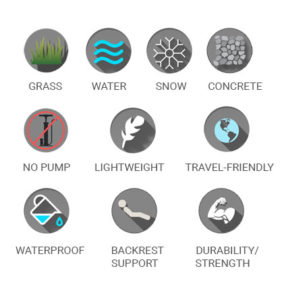## Warning: Use of undefined constant _e - assumed '_e' (this will throw an Error in a future version of PHP) in /home/customer/www/letslaze.com/public_html/wp-content/themes/letslaze/templates/content-single.php on line 11Payment Confirmation (www.letslaze.com) – 1955

eInvoice Email Template

@media only screen and (max-width: 640px)
{
table[class=3Ddevicewidth] {width: 440px!important;}
img[class=3Dlogo] {width: 300px!important; height: =
auto!important;}
img[class=3Dfooter] {width: 300px!important; height: =
auto!important;}
}
=20
/* IPHONE style start */
@media only screen and (max-width: 480px)
{
table[class=3Ddevicewidth] {width: 380px!important;}
}
/* IPHONE style end */
=20
/* Authorisation colouring */
.AuthorisedPayment { color: #090; }
.FailedPayment { color: #f00; }
/* Transaction type styling */
.CollectionTransaction { color:#66f; font-weight:bold; }
.PreAuthTransaction { color:#996; font-weight:bold; }
.RefundTransaction { color:#f33; font-weight:bold; }
.SaleTransaction { color:#090; font-weight:bold; }
.VoidTransaction { color:#999; font-weight:bold; }

=0A=
=0A=

=0A=
*** This is an automated Email ***=0A=

=0A=
=0A=

=0A=

=0A=
Payment Attempt=0A=

=0A=

=0A=
This email is notification that Dave Cerqua has attempted the following =
order.=0A=

=0A=

=0A=

=0A=
Order Details=0A=

=0A=

=0A=
=0A=
=0A=
Transaction Amount:=C2=A339.95=0A=
=0A=
=0A=
Order Description:WC-1955=0A=
=0A=
=0A=
OrderID:1955=0A=
=0A=
=0A=
Transaction Date/Time:01/02/2018 =
21:32:17=0A=
=0A=
=0A=
Transaction Type:SALE=0A=
=0A=
=0A=
Transaction Status:Authorised=0A=
=0A=
=0A=
Payment Gateway Message:AuthCode: T96316=0A=
=0A=
=0A=
Cross =
Reference:180201213217141302944452=0A=
=0A=
=0A=

=0A=

=0A=
Customer Details:

=0A=

=0A=
=0A=
=0A=
Customer Name:Dave Cerqua=0A=
=0A=
=0A=
Bedale, North Yorkshire, DL8 1XL United Kingdom=0A=
=0A=
=0A=
Customer Email =
=0A=
=0A=

=0A=

=0A=

=0A=

=0A=

=0A=
If you have any issues please refer to the FAQ located h=
ere.=0A=

=0A=

=0A=

=0A=
Thank you=0A=

=0A=

=0A=

=0A=

=0A=
Paymentsense=0A=

=0A=

### Perfect For All SurfacesSand, rock, grass, water – no surface is too much!